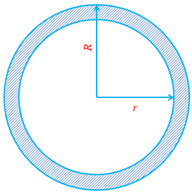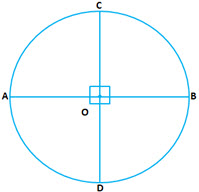Circumference of a circle: This is the length of the line that marks out the circle. We can also call it perimeter of the circle.

Circumference$(C) = 2 \pi r$  where$r =$ Radius of the circle.

(Note: Area of a circle$= \pi r^2$)A Circular Ring: This is the object that is bound by the circumference of two concentric circles of different radius.

If the radius of the outer ring is R and the radius of the inner ring is r, then the

Area of the ring$= \pi (R^2 - r^2)$

Semi-circle: If the circle is divided into two equal halves, then we will we will get two semi-circle.Length if the arc$= \pi r$ (half the circumference of the circle)

Perimeter of the semi-circle$= \pi r + 2r$$\displaystyle \text{Area of the semi circle } = \frac{1}{2} \pi r^2$Quadrant: If a circle is divided into four equal parts by two mutually perpendicular diameters,  the part so obtained is called a quadrant.$\displaystyle \text{Length of the arc APC } = \frac{1}{2} \pi r$

(one fourth of the circumference of the circle)$\displaystyle \text{Perimeter of the quadrant } = \frac{1}{2} \pi r + 2 r$$\displaystyle \text{Area of the quadrant } = \frac{1}{4} \pi r^2$